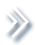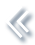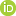####ArchivesReview Articles

## The effects of additive outliers in INAR(1) process and robust estimation

Marcelo Bourguignon ,

Departamento de Estatística, Universidade Federal do Rio Grande do Norte, Natal, RN, Brazilm.p.bourguignon@gmail.com

Klaus L. P. Vasconcellos

Departamento de Estatística, Cidade Universitária, Universidade Federal de Pernambuco, Recife, PE, BrazilPages 206-214 | Received 20 Jun. 2018, Accepted 03 Sep. 2018, Published online: 11 Sep. 2018,
• Abstract
• References
• Citations

ABSTRACT

In this paper, methods based on ranks and signs for estimating the parameters of the first-order integer-valued autoregressive model in the presence of additive outliers are proposed. In particular, we use the robust sample autocorrelations based on ranks and signs to obtain estimators for the parameters of the Poisson INAR(1) process. The effects of additive outliers on the estimates of parameters of integer-valued time series are examined. Some numerical results of the estimators are presented with a discussion of the obtained results. The proposed methods are applied to a dataset concerning the number of different IP addresses accessing the server of the pages of the Department of Statistics of the University of Würzburg. The results presented here give motivation to use the methodology in practical situations in which Poisson INAR(1) process contains additive outliers.

## References

1. Al-Osh, M. A., & Alzaid, A. A. (1987). First-order integer valued autoregressive (INAR(1)) process. Journal of Time Series Analysis8, 261–275. doi: 10.1111/j.1467-9892.1987.tb00438.x [Google Scholar]
2. Blomqvist, N. (1950). On a measure of dependence between two random variables. The Annals of Mathematical Statistics21, 593–600. doi: 10.1214/aoms/1177729754 [Google Scholar]
3. Boudt, K., Cornelissen, J., & Croux, C. (2012). The Gaussian rank correlation estimator: Robustness properties. Statistics and Computing22, 471–483. doi: 10.1007/s11222-011-9237-0 [Google Scholar]
4. Bourguignon, M., & Vasconcellos, K. L. P. (2015). Improved estimation for Poisson INAR(1) models. Journal of Statistical Computation and Simulation85, 2425–2441. doi: 10.1080/00949655.2014.930862 [Taylor & Francis Online], [Google Scholar]
5. Brännäs, K. (1994). Estimation and testing in integer-valued AR(1) models. Umeå Economic Studies335. Retrieved from http://www.usbe.umu.se/digitalAssets/39/39107_ues335.pdf [Google Scholar]
6. Brännäs, K., Hellströ, J., & Nordström, J. (2002). A new approach to modelling and forecasting monthly guest nights in hotels. International Journal Forecasting18, 19–30. doi: 10.1016/S0169-2070(01)00104-2 [Google Scholar]
7. Croux, C., & Dehon, C. (2010). Influence functions of the Spearman and Kendall correlation measures. Statistical Methods and Applications19, 497–515. doi: 10.1007/s10260-010-0142-z [Google Scholar]
8. Denby, L., & Martin, R. D. (1979). Robust estimation of the first order autoregressive parameter. Journal of the American Statistical Association74, 140–146. doi: 10.1080/01621459.1979.10481630 [Taylor & Francis Online], [Google Scholar]
9. Du, J., & Li, Y. (1991). The integer valued autoregressive (INAR(p)) model. Journal of Time Series Analysis12, 129–142. doi: 10.1111/j.1467-9892.1991.tb00073.x [Google Scholar]
10. Fajardo, F., Reisen, V. A., & Cribari-Neto, F. (2009). Robust estimation in long-memory processes under additive outliers. Journal of Statistical Planning Inference139, 2511–2525. doi: 10.1016/j.jspi.2008.12.014 [Google Scholar]
11. Fox, A. J. (1972). Outliers in time series. Journal of the Royal Statistical Society B34, 350–363. [Google Scholar]
12. Franke, J., & Seligmann, T. (1993). Conditional maximum-likelihood estimates for INAR(1) processes and their application to modelling epileptic seizure counts. In T. Subba Rao (Ed.), Developments in time series (pp. 310–330). London: Chapman & Hall. [Google Scholar]
13. Freeland, R. K., & McCabe, B. P. M. (2004). Analysis of low count time series data by Poisson autoregression. Journal of Time Series Analysis25, 701–722. doi: 10.1111/j.1467-9892.2004.01885.x [Google Scholar]
14. Freeland, R. K., & McCabe, B. P. M. (2005). Asymptotic properties of CLS estimators in the Poisson AR(1) model. Statistics and Probability Letters73, 147–153. doi: 10.1016/j.spl.2005.03.006 [Google Scholar]
15. Hájek, J., & Sidak, Z. (1967). Theory of rank tests. New York, NY: Academic Press. [Google Scholar]
16. Hellström, J. (2001). Unit root testing in integer-valued AR(1) models. Economics Letters70, 9–14. doi: 10.1016/S0165-1765(00)00344-X [Google Scholar]
17. Ispány, M., Barczy, M., Pap, G., Scotto, M., & Silva, M. E. (2010). Innovational outliers in INAR(1) models. Communications in Statistics – Theory and Methods39, 3343–3362. doi: 10.1080/03610920903259831 [Taylor & Francis Online], [Google Scholar]
18. Ispány, M., Barczy, M., Pap, G., Scotto, M., & Silva, M. E. (2011). Additive outliers in INAR(1) models. Statistical Papers53, 935–949. [Google Scholar]
19. Jung, R. C., Ronning, G., & Tremayne, A. R. (2005). Estimation in conditional first order autoregression with discrete support. Statistical Papers46, 195–224. doi: 10.1007/BF02762968 [Google Scholar]
20. Kachour, M., & Yao, J. F. (2009). First-order rounded integer-valued autoregressive (RINAR(1)) process. Journal of Time Series Analysis30, 417–448. doi: 10.1111/j.1467-9892.2009.00620.x [Google Scholar]
21. Kendall, M. G. (1938). A new measure of rank correlation. Biometrika30, 81–93. doi: 10.1093/biomet/30.1-2.81 [Google Scholar]
22. Li, Q., Lian, H., & Zhu, F. (2016). Robust closed-form estimators for the integer-valued GARCH(1,1) model. Computational Statistics and Data Analysis101, 209–225. doi: 10.1016/j.csda.2016.03.006 [Web of Science ®], [Google Scholar]
23. Martin, R. D., & Yohai, V. J. (1985). Robustness in time series and estimating ARMA models. In E. J. Hannan et al. (Eds.), Handbook of statistics (Vol. 5, p. 119–155). Science Publishers B.V. New York. [Google Scholar]
24. Park, Y., & Oh, C. W. (1997). Some asymptotic properties in INAR(1) processes with Poisson marginals. Statistical Papers38, 287–302. doi: 10.1007/BF02925270 [Google Scholar]
25. Sarnaglia, A. J. Q., Lévy-Leduc, V. A., & Reisen, C. (2010). Robust estimation of periodic autoregressive processes in the presence of additive outliers. Journal of Multivariate Analysis101, 2168–2183. doi: 10.1016/j.jmva.2010.05.006 [Google Scholar]
26. Schweer, S., & Weiß, C. (2014). Compound Poisson INAR(1) processes: Stochastic properties and testing for overdispersion. Computational Statistics and Data Analysis77, 267–284. doi: 10.1016/j.csda.2014.03.005 [Google Scholar]
27. Scotto, M. G., Weiß, C. H., & Gouveia, S. (2015). Thinning-based models in the analysis of integer-valued time series: A review. Statistical Modelling15, 590–618. doi: 10.1177/1471082X15584701 [Google Scholar]
28. Silva, M. E., & Pereira, I. (2015). Detection of additive outliers in Poisson INAR(1) time series. In Mathematics of Energy and Climate Change2, 377–388. doi: 10.1007/978-3-319-16121-1_19 [Google Scholar]
29. Silva, I, & Silva, M. E. (2018). Wavelet-based detection of outliers in Poisson INAR (1) time series. In Recent studies on risk analysis and statistical modeling. New York. Springer, 2018. [Google Scholar]
30. Spearman, C. (1904). General intelligence objectively determined and measured. The American Journal of Psychology15, 201–293. doi: 10.2307/1412107 [Google Scholar]
31. Steutel, F. W., & Van Harn, K. (1979). Discrete analogues of self-decomposability and stability. The Annals of Probability7, 893–899. doi: 10.1214/aop/1176994950 [Google Scholar]
32. Weiß, C. H. (2007). Controlling correlated processes of Poisson counts. Quality and Reliability Engineering International23, 741–754. doi: 10.1002/qre.875 [Google Scholar]
33. Weiß, C. H. (2008). Thinning operations for modelling time series of counts - a survey. Advances in Statistical Analysis92, 319–341. doi: 10.1007/s10182-008-0072-3 [Google Scholar]
34. Weiß, C. H. (2011). Simultaneous confidence regions for the parameters of a Poisson INAR(1) model. Statistical Methodology8, 517–527. doi: 10.1016/j.stamet.2011.07.003 [Google Scholar]
35. Weiß, C. H. (2012). Process capability analysis for serially dependent processes of Poisson counts. Journal of Statistical Computation and Simulation82, 383–404. doi: 10.1080/00949655.2010.533178 [Taylor & Francis Online], [Google Scholar]
36. Zeger, S. L., & Qaqish, B. (1988). Markov regression models for time series: a quasi-likelihood approach. Biometrics44, 1019–1031. doi: 10.2307/2531732 [Google Scholar]
37. Zhu, F. (2012a). Modeling overdispersed or underdispersed count data with generalized Poisson integer-valued GARCH models. Journal of Mathematical Analysis and Applications389, 58–71. doi: 10.1016/j.jmaa.2011.11.042 [Google Scholar]
38. Zhu, F. (2012b). Modeling time series of counts with COM-Poisson INGARCH models. Mathematical and Computer Modelling56, 191–203. doi: 10.1016/j.mcm.2011.11.069 [Google Scholar]